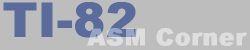Lesson 14
 TI-82 ASM Corner :: Programming Guide :: Lesson 14 Back | Home | Search
Lesson 14 : Bit manipulation, shifting and rotating

In this lesson, you will learn how to manipulate the contents of a byte by shifting or rotating it. You will also learn how to test and change the contents of a single bit.

shift instructions | rotate instructions | bit manipulation

Shift instructions

First of all, what do I mean with shifting? Well, it means that you move all the bits of a byte either one bit to the left or one bit to the right. The bit that falls out will end up in the carry flag and according to the type of instruction, the empty bit will be reset or left unchanged.

SLA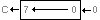Shift Left Arithmeticly, shifts the byte 1 bit to the left and inserts 0 at the empty position on the right. This is often used to multiply a register with 2. SLA B would store B*2 into the B register. SLA A also works, but it's better to use ADD A,A instead, since it is faster and takes onlu one byte.

SRA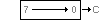Shift Right Arithmeticly, shifts the byte 1 bit to the right and does not change bit 7. This shift can be useful when you are using 2's complement for negative numbers. More about 2's complement in one of the following lessons.

SRL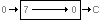Shift Right Logically, shifts the byte 1 bit to the right and resets bit 7. This instruction is often used to divide a byte by 2. The remainder is then stored in the carry flag.

Rotate instructions

First, what is rotating? Well, rotating is very similar to shifting, but instead of putting a zero at the end/beginning (except SRA), the carry flag will be inserted there. This way you will get the original byte back when you rotate 8 or 9 times. There are 10 different rotate instructions. Here they come:

RL and RLA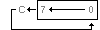Rotate Left (Accumulator). With RL, you have to specify which register you want to rotate. RLA is the same as RL A and it was included because of backwards compatibility with the 8080 processor.
There's a little difference between RL A and RLA when talking about the flags, the size of the instruction and the speed. With RLA the zero flag is left unchanged while RL A affects the zero flag. RLA takes one byte while RL A takes 2 and RLA is a little faster. This instruction is an 9 bit rotation.

RLC and RLCA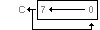Rotate Left Circular (Accumulator).
Once again, RLCA does the same as RLC A. The differences are the same as above. This is a 8 bit rotation. Instead of rotating through the carry, bit 7 is transferred immediately to bit 0, but bit 7 is also copied into the carry flag so you can easily check what it was.

RR and RRA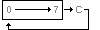Rotate Left (Accumulator).
This works in the same way as RL and RLA, but instead of rotating to the left, RR rotates to the right.

RRC and RRCA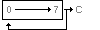Rotate Right Circular (Accumulator).
And this one is the same as RLC and RLCA, but this time rotating is done to the right.

RLD and RRD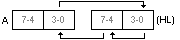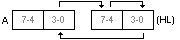Rotate Digit Left and Rotate Digit Right This instruction is not important when programming games, but I will tell you how it works anyway.
This instruction rotates the lower nibble of the accumulator to the upper nibble of (HL), the upper nibble of (HL) to the lower nibble of (HL) and the lower nibble of (HL) back to the lower nibble of the A register (that's for the RRD instruction). This is useful when you are dealing with BCD numbers. This is a method to store numbers. Each nibble represents a digit then. e.g. 93 would be something like \$93.

Bit manipulation

There are 3 instructions that let you change or test a specific bit in a byte: SET, RES and BIT. You probably still know that the 8 bits from a byte are numbered 0-7 from right to left (lower bit to upper bit).

BIT b,m

This instruction tests a bit. Actually it copies the contents of the bit to the zero flag. This means that is the bit was set, the zero flag will also be set and if the bit was not set, the zero flag will be reset. This instruction does not change anything to the byte you tested.
b indicates the number of the bit (0-7)
m can be A, B, C, D, E, H, L, (HL), (IX+n), (IY+n)

SET b,m

This instruction sets a bit. It changes the selected bit to 1 and doesn't affect the flags.
b indicates the number of the bit (0-7)
m can be A, B, C, D, E, H, L, (HL), (IX+n), (IY+n)

RES b,m

This instruction resets a bit. It changes the selected bit to 0 and doesn't affect the flags.
b indicates the number of the bit (0-7)
m can be A, B, C, D, E, H, L, (HL), (IX+n), (IY+n)

New instructions

Here is a little table with all the new instructions from this lesson.

New instructions
Mnemonic Operation
SLA m Shift Left Arithmetically
m can be r, (HL), (IX+n), (IY+n)
all flags affected
SRA m Shift Right Arithmetically
m can be r, (HL), (IX+n), (IY+n)
all flags affected
SRL m Shift Right Logically
m can be r, (HL), (IX+n), (IY+n)
all flags affected
RLCA Rotate Left Circular Accumulator
only CY flag affected
RLA Rotate Left Accumulator
only CY flag affected
RRCA Rotate Right Circular Accumulator
only CY flag affected
RRA Rotate Right Accumulator
only CY flag affected
RLC m Rotate Left Circular
m can be r, (HL), (IX+n), (IY+n)
Zero, Sign and Carry affected
RL m Rotate Left
m can be r, (HL), (IX+n), (IY+n)
Zero, Sign and Carry affected
RRC m Rotate Right Circular
m can be r, (HL), (IX+n), (IY+n)
Zero, Sign and Carry affected
RR m Rotate Right
m can be r, (HL), (IX+n), (IY+n)
Zero, Sign and Carry affected
RLD Rotate Digit Left
Only zero and sign flag affected
RRD Rotate Digit Right
Only zero and sign flag affected
BIT b,m Copy bit to zero flag (test bit)
m can be r, (HL), (IX+d), (IY+d)
b is bit number (0-7)
RES b,m Reset bit
m can be r, (HL), (IX+d), (IY+d)
b is bit number (0-7)
SET b,m Set bit
m can be r, (HL), (IX+d), (IY+d)
b is bit number (0-7)
r can be B, C, D, E, H, L, A
n is an 8-bit value in range 0-255

Previous lesson | Contents | Next lesson